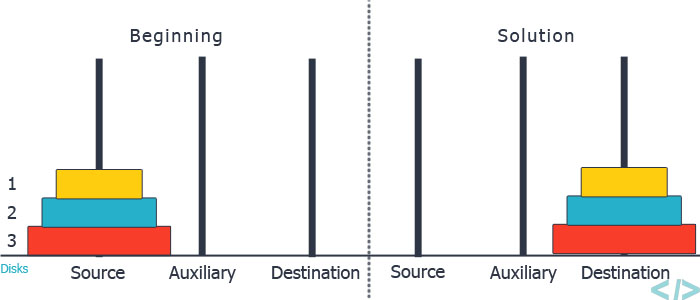New update is available. Click here to update.

# Tower of Hanoi

Last Updated: 23 Feb, 2023
Easy0/40
Avg time to solve 15 mins
Success Rate 85 %Share125 upvotes

## Problem Statement

#### You are given three rods (numbered 1 to 3), and ‘N’ disks initially placed on the first rod, one on top of each other in increasing order of size ( the largest disk is at the bottom). You are supposed to move the ‘N’ disks to another rod(either rod 2 or rod 3) using the following rules and in less than 2 ^ (N) moves.

``````1. You can only move one disk in one move.
2. You can not place a larger disk on top of a smaller disk.
3. You can only move the disk at the top of any rod.
``````
##### Note :
``````You may assume that initially, the size of the ‘i’th disk from the top of the stack is equal to ‘i’, i.e. the disk at the bottom has size ‘N’, the disk above that has size ‘N - 1’, and so on. The disk at the top has size 1.
``````
##### Example :Detailed explanation ( Input/output format, Notes, Images )##### Constraints :
``````1 <= T <= 5
1 <= N <= 12

Where ‘T’ denotes the number of test cases, ‘N’ denotes the number of disks.

Time Limit: 1 sec
``````
##### Sample Input 1 :
``````2
1
2
``````
##### Sample Output 1 :
``````1
1
``````
##### Explanation of Sample Input 1 :
``````In the first test case,
you can move the only disk to either rod 2 or rod 3. The matrix to be returned should be either { { 1, 2 } } or { {1, 3 } }.

In the second test case,
you can move the topmost disk from rod 1 to rod 2. Then move the remaining disk from rod1 to rod 3. Now move the disk in rod 2 to rod 3. . One of the correct ways to return the output is { { 1, 2 }, { 1, 3 }, { 2, 3 } }.
``````
##### Sample Input 2 :
``````1
3
``````
##### Sample Output 2 :
``````1
``````
##### Explanation of Sample Input 2 :
``````One of the correct matrices is { { 1, 2 }, { 1, 3 }, { 2, 3 }, { 1, 2 }, { 3, 1 }, { 3, 2 }, { 1, 2 } }.
``````AutoConsole